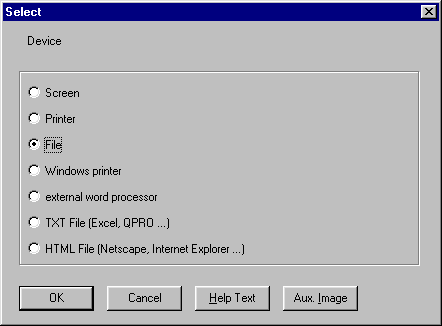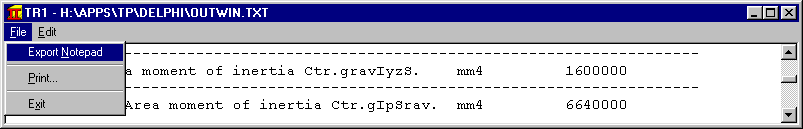English Deutsch

# Info Letter No. 64 - Nov./December 2000

### Copyright by HEXAGON Software 2000

#### by Fritz Ruoss

WN4 - Software for Involute Splines to ANSI B92.1

The new WN4 software calculates involute splines according to ANSI B92.1 and ANSI B92.1b.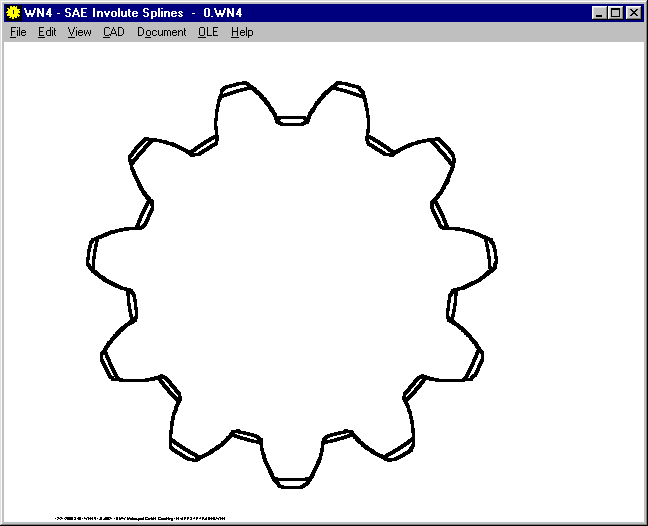Pressure Angle of involute splines to SAE may be 30, 37.5 or 45 degrees. Normal circular pitch ranges from 2.5 / 5 to 128 / 256.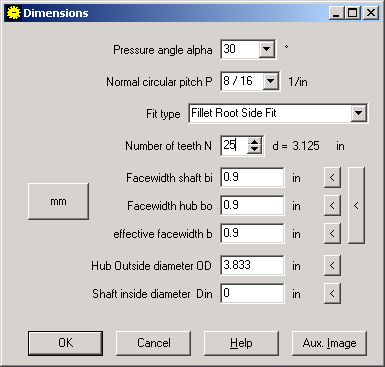Spline Tolerance class can be selected from 4 to 7. If you choose calculation to ANSI B92.1b, the fit class can be selected as well.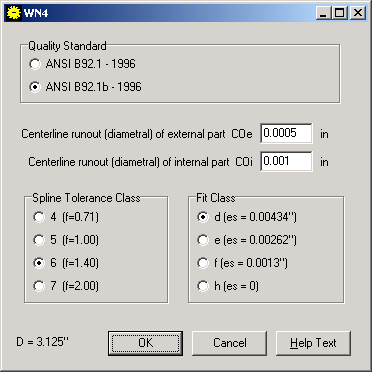WN4 calculates tooth thickness and dimensions over pins. Pin diameter can be changed.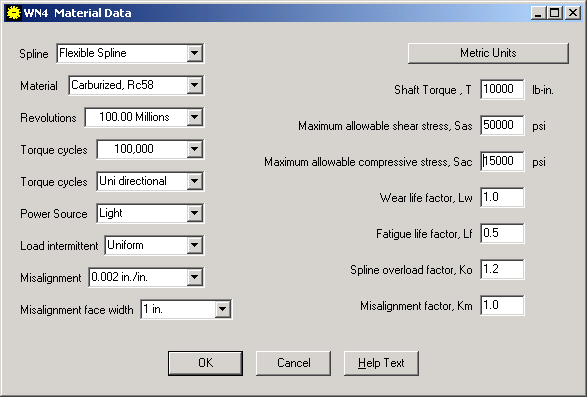WN4 calculates compressive stress, spline teeth shear stress, hoop stress, bending stress, torsional stress, and equivalent stress according to "SAE Design Guide for Involute Splines". Material, application factors and life expectation coefficients can be entered directly, or calulated by WN4.

FED1+ Calculation Options

The options for spring calculation are all in one dialogue windows now, so you can set/reset different calculation options very fast.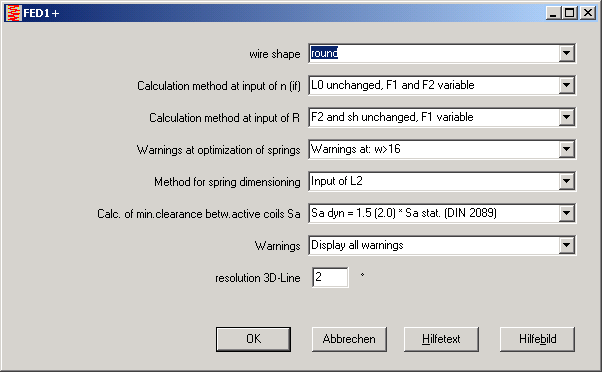FED2+ Manufacturing Compensation

Manufacturing tolerances are to be compensated by F0 instead of L0. Thanks to Mr. Heilberg of Stahlschmidt for this hint.

SR1 ñ Tightening torque

MA is the maximum tightening torque according to VDI 2230. The minimum torque is MA/alphaA. So you must not set the tightening tool torque to MA, but to the average value of MA and MA/alphaA. The torque tolerance is based on the average value, it can be converted to alphaA with:

```
2 * alphaA
deviation [%] = ( ------------  -  1 ) * 100
1 + alphaA

100% + deviation[%]
alphaA = -------------------
100% - deviation[%]

```

Example: tightening tool tolerance is +/- 10%. alpha A is then 110/90 = 1.222. SR1 calculates MA=100 Nm. Minimum is MAmin= 81.8 Nm. The tightening tool has to be set to the average value M=90.9 Nm. With deviation of 10%, bolted joint will be tightened with M=90.9+/-9.1 Nm.

SR1 ñ Residual clamping force for pressure load

Residual clamping force is calculated with prestressing force FV ñ Suppl.plate force FPA. The critical interface is between the clamping plates. For real pressure load (FAo=0, Fau<0), or if the absolute value of the compression force is higher than the tractive force (Fau < -Fao), the critical interface is between screw head and first clamping plate.

Residual clamping force is then FKR = FV + FPA (for FPA < 0).

Formula for both, tension and pressure, are then (abs=absolute value):

```FKRo = FV + abs(FPAo)
FKRu = FV + abs(FPAu)
```

At SR1 Printout, some signs were changed: Fko min and Fku min were changed to FKRo and FKRu, the required residual clamping force is now Fkerf (instead of FKR).

WL1+ DIN 743

At "View-Position", you can show the stress values and coefficients for any x-position of the shaft. The new version also prints strength values and coefficients, average stresses and amplitudes, if DIN 743 was selected as calculation option.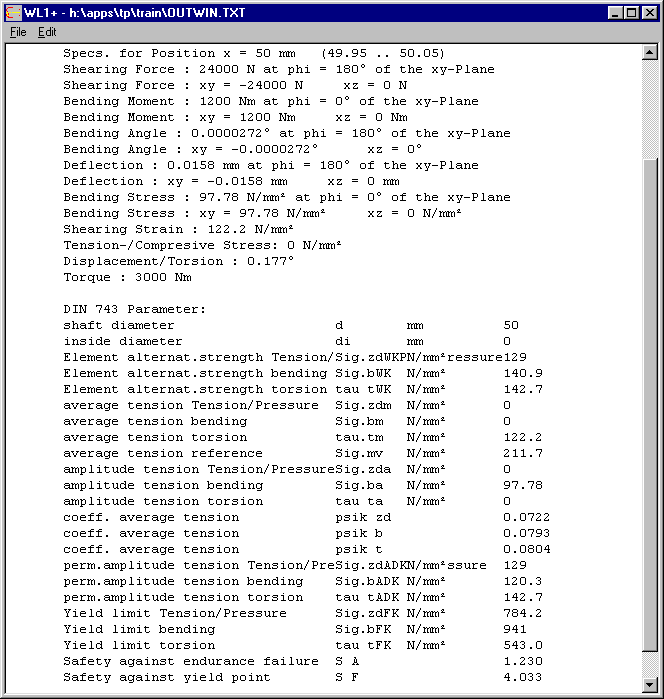TR1 - 3D

A 3D-drawing of the girder can be displayed on screen now.ZAR1+ Pre-Dimensioning

The input values for pre-dimensioning of a gear are all on one dialogue window now. If you you use the "<" buttons for suggestions, you must begin on top (with the pressure angle) and then enter the next values, because suggestions use the upper values that were entered before.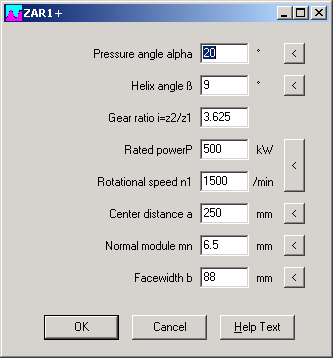ZAR1+ Dimension Input

The new button x1<->x2 swaps addendum modification coefficients of pinion and gear 2. Another improvement of the dimension window is the higher precision up to 5 decimals.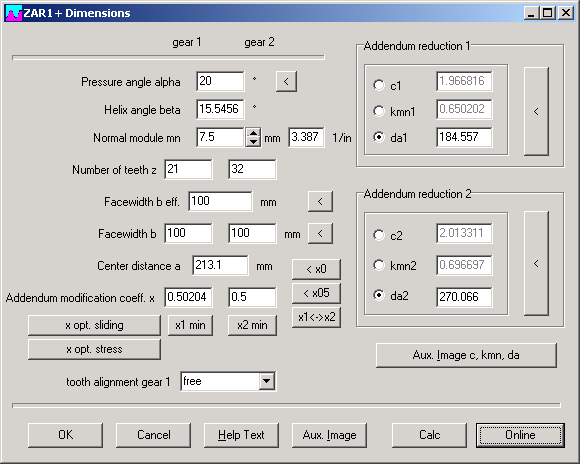Configuration - 3D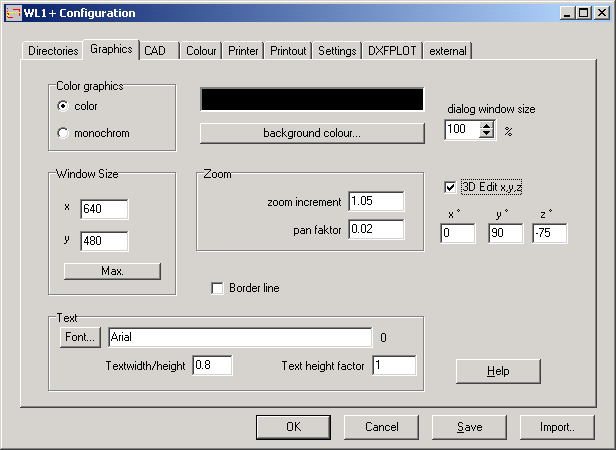The former 3D drawing started with a dialogue window for x,y,z angle to be edited. Now you can configure the default 3D angles of the coordinates, and define if the dialogue window should be displayed or not.

Printout -> File

If you print to file, 2 files are generated:

1. A text file of MS-DOS 8-bit format
2. A text file OUTWIN.TXT of Windows format

If you want to import the Windows file, save OUTWIN.TXT with another filename, or use the new "Export Notepad" funktion at Screen Device.